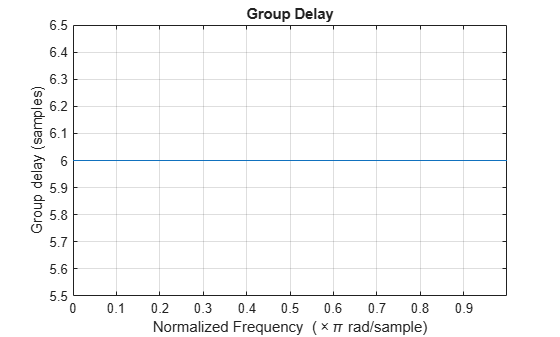# grpdelay

Group delay response of discrete-time filter System object

## Syntax

``````[gd,w] = grpdelay(sysobj)``````
``````[gd,w] = grpdelay(sysobj,n)``````
``````[gd,w] = grpdelay(sysobj,'Arithmetic',arithType)``````
``grpdelay(sysobj)``

## Description

``````[gd,w] = grpdelay(sysobj)``` returns the group delay `gd` of the filter System object™, `sysobj`, based on the current filter coefficients. The vector `w` contains the frequencies (in radians) at which the group delay is evaluated. The group delay is defined as: $-\frac{d}{dw}\left(angle\left(w\right)\right)$The group delay is evaluated at 8192 points equally spaced around the upper half of the unit circle.```
``````[gd,w] = grpdelay(sysobj,n)``` returns the group delay of the filter System object and the corresponding frequencies at `n` points equally spaced around the upper half of the unit circle.```
``````[gd,w] = grpdelay(sysobj,'Arithmetic',arithType)``` computes the group delay of the filter System object, based on the arithmetic specified in `arithType`, using either of the previous syntaxes.```

example

````grpdelay(sysobj)` plots the group delay of the filter System object in the `fvtool`.For more input options, see `grpdelay` in Signal Processing Toolbox™.```

## Examples

collapse all

`CICComp = dsp.CICCompensationDecimator;`

`grpdelay` computes the group delay of the filter and displays it using `fvtool`.

`grpdelay(CICComp);`## Input Arguments

collapse all

Number of samples, specified as a positive integer. For an FIR filter where `n` is a power of two, the computation is done faster using FFTs.

Data Types: `single` | `double` | `int8` | `int16` | `int32` | `int64` | `uint8` | `uint16` | `uint32` | `uint64`

Arithmetic used in the filter analysis, specified as `'double'`, `'single'`, or `'Fixed'`. When the arithmetic input is not specified and the filter System object is unlocked, the analysis tool assumes a double-precision filter. When the arithmetic input is not specified and the System object is locked, the function performs the analysis based on the data type of the locked input.

The `'Fixed'` value applies to filter System objects with fixed-point properties only.

When the `'Arithmetic'` input argument is specified as `'Fixed'` and the filter object has the data type of the coefficients set to `'Same word length as input'`, the arithmetic analysis depends on whether the System object is unlocked or locked.

• unlocked –– The analysis object function cannot determine the coefficients data type. The function assumes that the coefficients data type is signed, has a 16-bit word length, and is auto scaled. The function performs fixed-point analysis based on this assumption.

• locked –– When the input data type is `'double'` or `'single'`, the analysis object function cannot determine the coefficients data type. The function assumes that the data type of the coefficients is signed, has a 16-bit word length, and is auto scaled. The function performs fixed-point analysis based on this assumption.

To check if the System object is locked or unlocked, use the `isLocked` function.

When the arithmetic input is specified as `'Fixed'` and the filter object has the data type of the coefficients set to a custom numeric type, the object function performs fixed-point analysis based on the custom numeric data type.

## Output Arguments

collapse all

Group delay vector of length `n`. If `n` is not specified, the function uses a default value of `8192`.

Data Types: `double`

Frequency vector of length `n`, in radians/sample. `w` consists of `n` points equally spaced around the upper half of the unit circle (from 0 to π radians/sample). If `n` is not specified, the function uses a default value of `8192`.

Data Types: `double`

### Topics

Introduced in R2011a

## Support평가판 신청